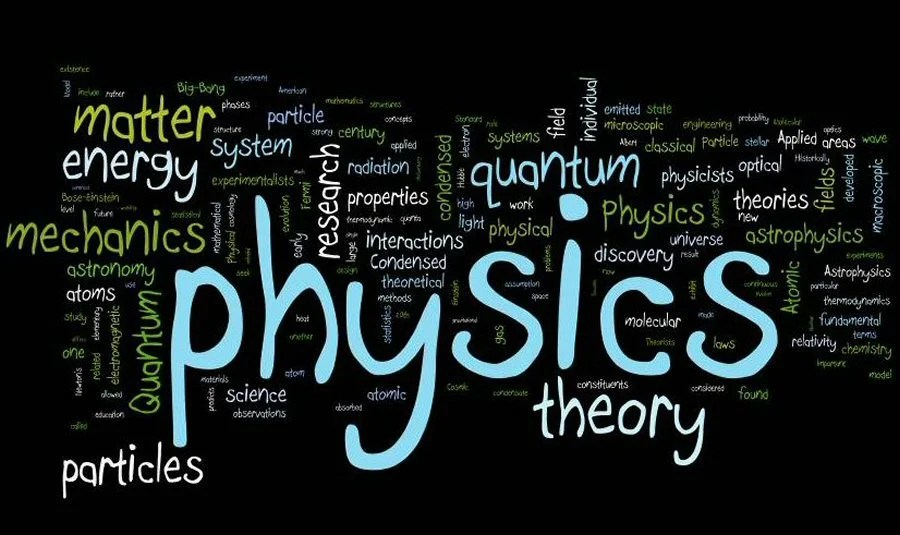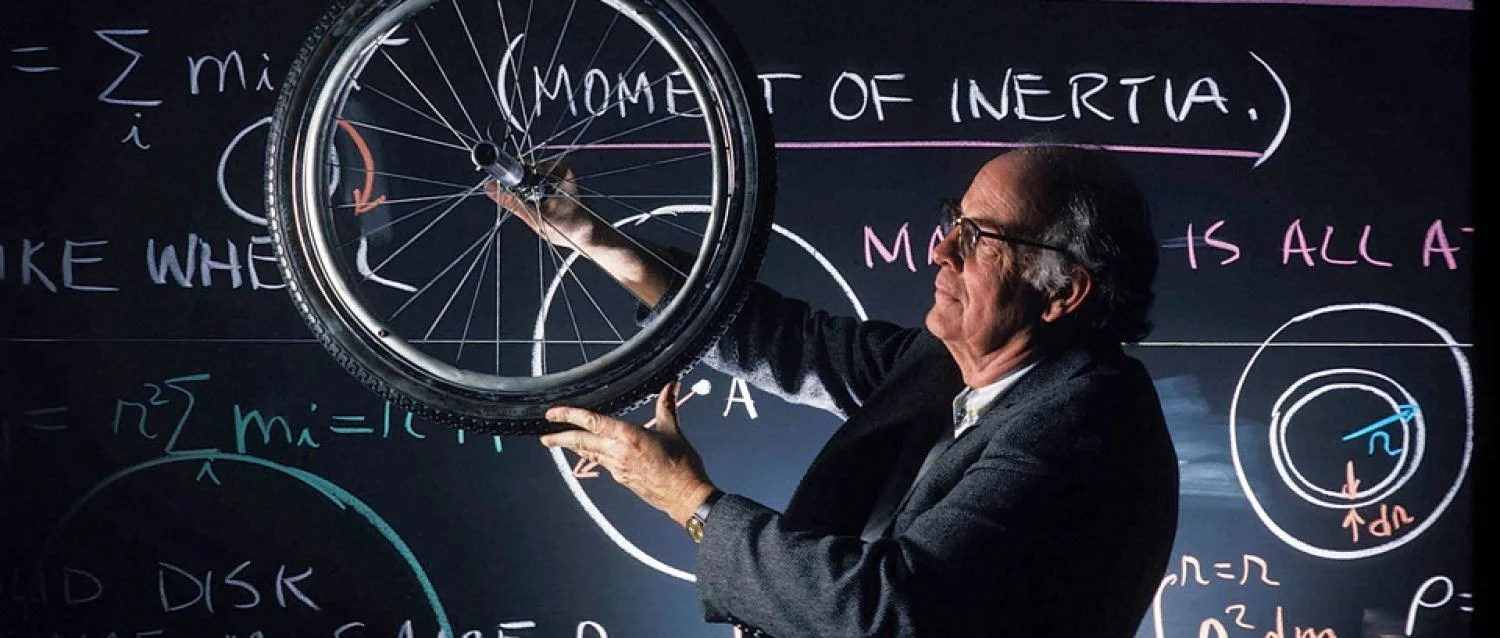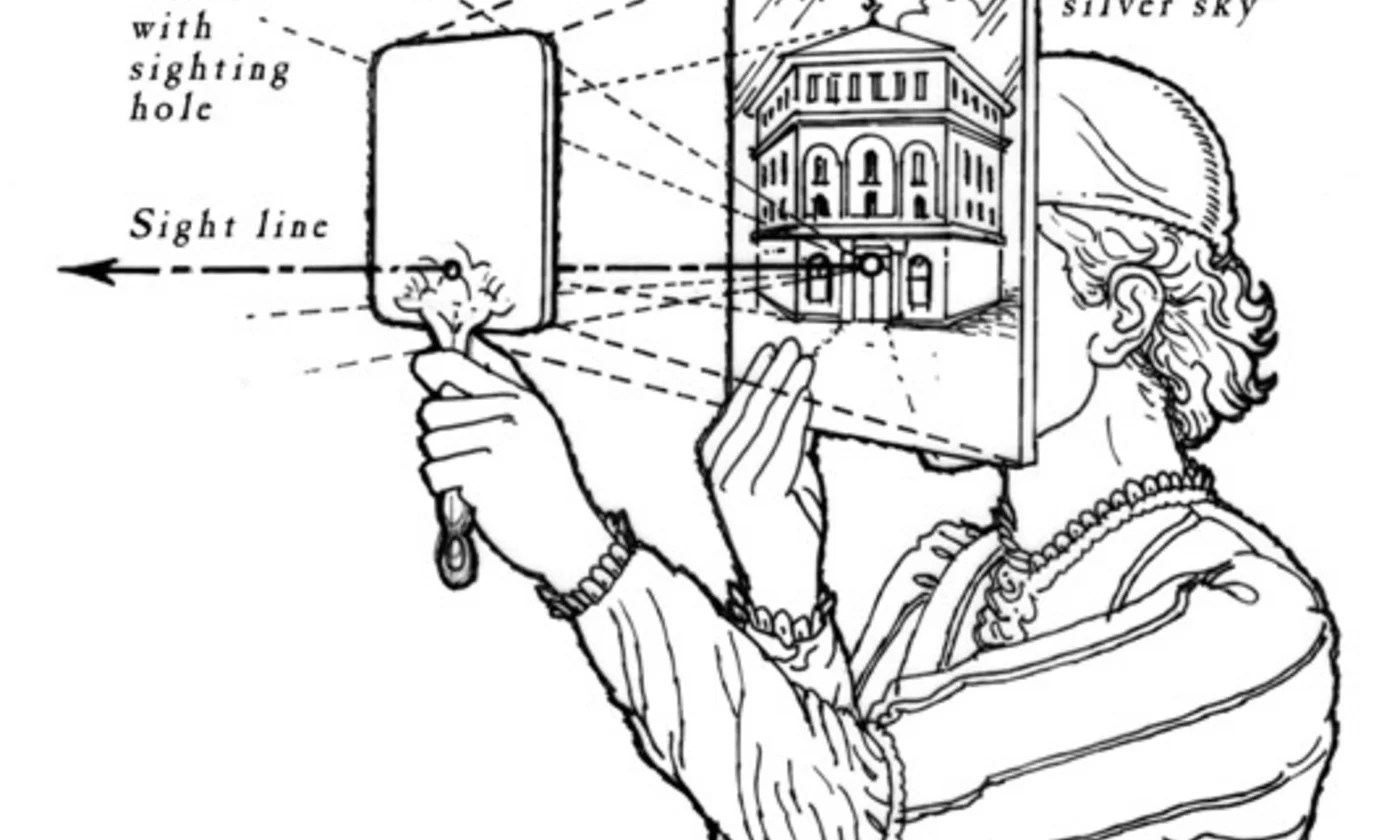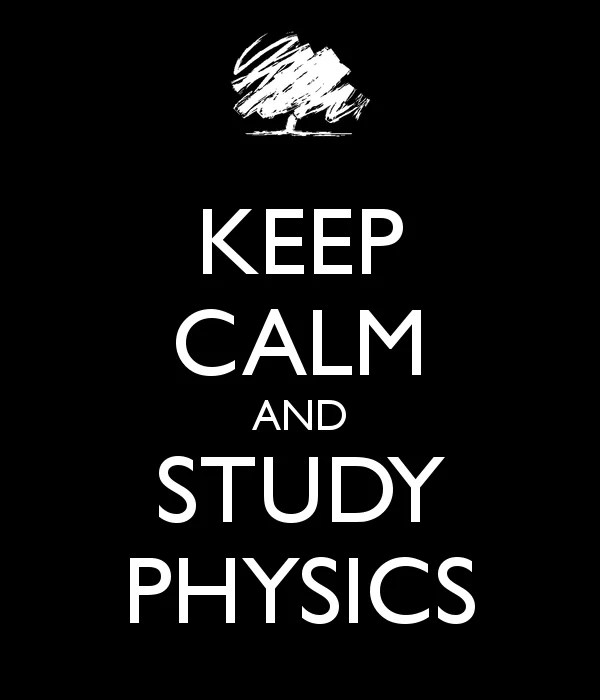# Physics, why and what?

For medical students as well as medical professionals, understanding physics helps tremendously in three major ways.First, it helps clarify the functionality of the medical sciences and technology. Second, physics helps explain the physical systems occurring in the body and, finally, understanding physics helps in many other fields providing the framework to solve unsolved problems.

Here are the key concepts that all the first year medical students must have in their minds before continuing to study Physiology, not to mention “in relation to chemistry and biology.”1. Motion
Motion is defined as the act of changing location or position. Three types of motion exist: translational, oscillatory, and rotational. Translational motions occur when a change in location takes place. Oscillatory motion is motion that takes place without changing location. An example of such motion is the vibration of strings on a musical instrument. Rotational motion deals with the spinning that occurs in objects.

2. Force
Force occurs when two objects interact with each other. Each object has a force on the other: a push or a pull. When the interaction between the two objects stops, then the force also disappears. This force can be separated into two categories: forces from contact and forces from action-at-a-distance. The difference between the two classifications is whether the two objects are in direct contact with each other or not. The unit of force is newton (N).

3. Equilibrium
The definition of equilibrium is defined in the word itself. It deals with equal parts implying a balance. If an object has forces acting on it, the object may undergo motion. However, if the object is in equilibrium, then the object will not move since all the forces balance each other.

One way to look at the motion of an object is to separate the forces acting on the object into horizontal and vertical forces. In equilibrium, the horizontal forces will balance themselves and the vertical forces will balance themselves; thus, no motion of the object will occur in either the horizontal or vertical axes.

4. Momentum
Momentum can be understood by looking at mass and velocity of an object. Mass is a property of an object that measures the amount of matter in an object. Velocity is the amount of distance an object travels over a given time. It is a vector quantity so directionality is also expressed. Momentum is the product of mass and velocity, also a vector quantity. In practicality, momentum refers to the quantity of motion possessed by an object. The unit of momentum is kilogram-meter per second (kg-m/s).

5. Energy
Energy is the property that is transferred to an object to allow it to generate work. Energy in the universe is fixed, implying that it can be transferred but cannot be created nor destroyed. Energy exists in many forms: kinetic (moving energy), potential (storing energy), elastic (stretching energy), chemical (reaction energy), radiant (light energy), and thermal (temperature energy). Energy stays constant overall as it is transferred from one form to another.

6. Work
Work is the result that takes place when a force is applied to an object to move the object. It can be measured by multiplying the force by the distance. Work is closely related to energy. The concept referred to as work-energy principle states that an increase in kinetic energy of an object is due to an equal amount of work performed on the object. The unit of work is the joule (J) which equals a newton-meter (N-m).7. Fluids
Fluids are one of the phases of matter that deform due to the application of pressure or stress. This capability allows fluids to travel through different channels in the body to carry substances such as oxygen, cells, waste, antibodies, and medicines. Fluids flow according to Bernoulli’s principle, which states that fluids increase their speeds as pressure decreases or the fluid’s potential energy decreases.

8. Gases
Gases are one of the phases of matter where the particles are vastly separated unlike what takes place in solids and liquids where particles are closer together. Gases are hard to observe so they are classified according to temperature, number of particles, pressure, and volume.

Gases have low density and viscosity compared to other states of matter. Two laws explain their properties. Boyle’s law states that at a constant temperature, the pressure of the gas varies inversely with its volume. Charles’s law states that at a constant pressure, the volume of the gas is directly proportional to its temperature. These laws explain the movement of gases in different body compartments.

9. Periodic motion
Periodic motion is motion that occurs in equal intervals of time. It can be seen in a rocking chair, in a swing in motion, in a planet orbiting the sun, a vibrating tuning fork, and a water wave. The period is the interval of time for the cycle to take place. It can be explained by the term frequency, which refers to the number of periods per time unit.

10. Electricity
Electricity is associated with the existence of an electrical charge dependent on the number of electrons, the negative particle in an atom. When the electrical charges are not moving, it is referred to as static electricity. If electrons are moving, it is referred to as dynamic electricity.

In the human body, different ions (positively or negatively charged substances) can move based on electrical differences that exist across membranes. This allows for the transportation of substances and conduction of impulses needed for nerve and muscle transmission.11. Magnetism
Magnetism is the physical action that takes place arising from the force caused by magnets (objects that generate fields that attract or repel other objects). The particles that undergo this effect are electrically charged particles. The force that is created depends on the charge magnitude, the particle velocity, and the magnetic field strength. The MRI (magnetic resonance imaging) generates pictures of the anatomy and physiology of the body using magnetic fields.

12. Circuits
A circuit is a closed loop through which charges can continuously move. For instance, when you turn on the light switch, the light turns on. The switch is acting to close the circuit allowing electrons to move and be transformed into light energy. When the circuit is closed, the electron motion is referred to as the current of the circuit. The human body has many circuits where electrical activity generates physiologic activity associated with nerve transmission and muscle contraction.

13. Waves
A wave is a disturbance that travels through a medium from one place to another place. What is a medium? A medium is the substance that carries the wave. In a pond that is undisturbed, the water is quiet in its resting position with a smooth surface. When a rock is dropped, a disturbance is generated. The change that occurs from the rock causes a fluctuation in the water which is transmitted as a wave that travels either to the shore or disperses over time. In the body, fluid travels in channels with a wave effect.

14. Light
Light is electromagnetic radiation that can be detected by the human eye. Electromagnetic radiation is the flow of energy through space and a material medium in form of electric and magnetic fields. The elementary particle of light is a photon. Visible light reaches the visual layer of the eye whereby it is converted to chemical energy and transmitted to the brain via nerve impulses. The brain reforms the image allowing sight.15. Sound
Sound is a pressure or mechanical wave that is formed by the back and forth vibration of particles. The wave leaves the source in all directions and can be sensed by sensors. In humans, the sound waves are captured by the ear and transmitted into the ear canal creating vibratory motion of the middle ear bones. The waves that are generated by vibration are longitudinal waves.

16. Atoms
An atom is the smallest particle into which an element can be divided without losing its chemical identity. An atom is made up of electrons, protons, and neutrons. The central nucleus is made up of the neutrally charged neutrons and the positively charged protons. The negatively charged electrons exist as a cloud orbiting the nucleus. The electron configuration and number determine the ability for elements to bond creating chemical reactions.

17. Electronic structure
The electronic structure or configuration is the arrangement of electrons in energy levels around the central nucleus in an atom. The levels are different orbits in which the electrons exist. The electrons fill the orbits closer to the nucleus and, depending on the element, extend the orbitals outward. The ability for elements to bind is based on the status of the outer electron shell.18. Thermodynamics
Thermodynamics is associated with heat and temperature and their association to energy and work. Various laws exist that define these relationships.

The first law states that the internal energy of an isolated system is constant. An isolated system does not exchange heat, work, or matter with the surroundings.

The second law states that heat cannot spontaneously flow from a colder location to a hotter location.

The third law states that all processes cease as a system approaches absolute zero.

19. Thermochemistry
Thermochemistry deals with the energy and heat and their association with chemical relations and physical transformations. Reactions can either absorb or release energy. It also explains what occurs during phase changes, such as melting and boiling. The human body performs chemical reactions, which are classified as endothermic and exothermic. Endothermic reactions absorb heat, whereas exothermic reactions release heat.